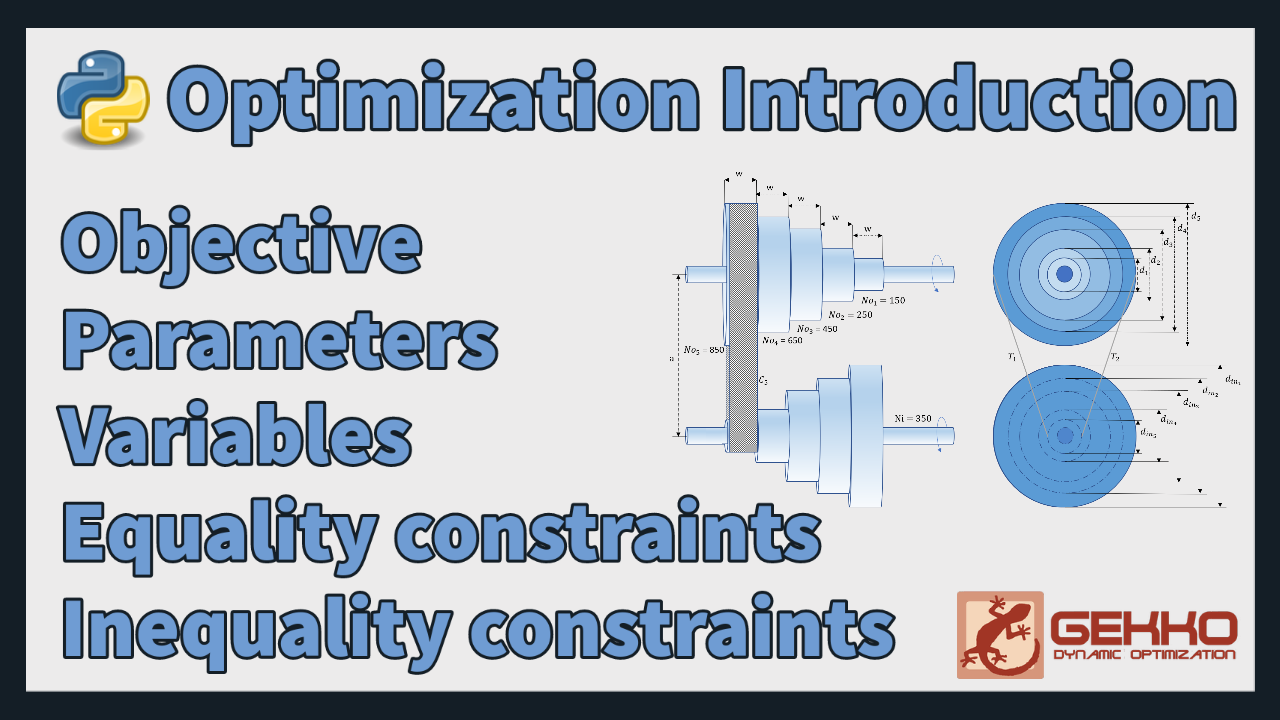## Optimization Introduction

Engineering optimization platforms in Python are an important tool for engineers in the modern world. They allow engineers to quickly and easily optimize complex engineering problems and tasks, such as design optimization, resource allocation, and route planning. Engineering optimization platforms typically offer powerful algorithms, such as constraint satisfaction algorithms, genetic algorithms, or artificial neural networks, to help engineers find the best solutions to their problems. Additionally, many engineering optimization platforms also provide tools for data visualization and interactive exploration, allowing engineers to gain a better understanding of their optimization results.

Python Gekko Example

The following is an example of using Python Gekko to solve an optimization problem in Python. The problem is to minimize the cost of a product given the cost of the three components that are required to produce the product.

1️⃣ Import Gekko and create a new model:

from gekko import GEKKO
m = GEKKO()

2️⃣ Next, define the variables to be optimized. In this problem, there are three components with prices x1, x2, and x3 that have lower (lb) and upper (ub) bounds.

x1 = m.Var(value=2,lb=0,ub=2)
x2 = m.Var(value=3,lb=0,ub=2)
x3 = m.Var(value=4,lb=0,ub=2)

3️⃣ Define an equation or other constraints:

m.Equation(x1 + x2 + x3 == 5)
m.Equation(x2 + x3 <= 4)

4️⃣ Define the objective function to minimize the cost of the product:

m.Minimize(0.5*x1 + 0.8*x2 + 0.7*x3)

5️⃣ Finally, solve the optimization problem and print the optimal values of the components:

m.solve(disp=False)
print('Optimal cost: ' + str(m.options.objfcnval))
print('x1: ' + str(x1.value))
print('x2: ' + str(x2.value))
print('x3: ' + str(x3.value))

The solution satisfies the constraints and minimizes the objective function.

    Optimal cost: 3.1999999556
x1: 2.0
x2: 0.99999994451
x3: 2.0


Because Python Gekko uses numerical methods, the solution is accepted within a specified tolerance that balances solution time with accuracy. The exact solution is x1=2, x2=1, x3=2 and optimal cost=3.2.

pip install gekko
from gekko import GEKKO
m = GEKKO()
x1 = m.Var(value=2,lb=0,ub=2)
x2 = m.Var(value=3,lb=0,ub=2)
x3 = m.Var(value=4,lb=0,ub=2)
m.Equation(x1 + x2 + x3 == 5)
m.Equation(x2 + x3 <= 4)
m.Minimize(0.5*x1 + 0.8*x2 + 0.7*x3)
m.solve(disp=False)

print('Optimal cost: ' + str(m.options.objfcnval))
print('x1: ' + str(x1.value))
print('x2: ' + str(x2.value))
print('x3: ' + str(x3.value))

Python Gekko is a package for optimization and testing of dynamic systems. It is a powerful open-source software package that allows users to easily solve complex nonlinear optimization problems. It is used in a variety of fields, including engineering, economics, finance, and operations research. Python Gekko provides a comprehensive suite of features and algorithms to help users solve difficult optimization problems with ease. It also has a user-friendly interface that makes it easy to quickly set up and solve problems. Python Gekko has been used in a variety of research fields, including energy systems, aerospace engineering, and machine learning. See additional Python Gekko tutorials and the introduction to optimization.

• Complete the information sheet to tell me a little more about your background and interests
• Think of an optimization problem. Define the following for this optimization problem of your choice:
• Objective
• Analysis variables (Parameters)
• Design variables (Variables)
• Equality constraints
• Inequality constraints
• Continuous variables
• Discrete variables
• Optimizers sometimes report that the optimization problem is infeasible. What does infeasible mean and how can it generally be corrected?
• Give the general form of the following types of problems and list at least one method that is used to solve them.
Example problems for each can be solved by clicking on the links and selecting the run button (green arrow). The solution appears in the window below the model file by selecting the results table icon.
• Define the following and tell how it is relevant to optimization:
• Review information on optimization platforms (2 or 3 is fine) such as:

See Optimization Software for a review of Open-Source Modeling Languages.

• What optimization software tutorials did you review?
• What resources (at least 3) did you find particularly useful or informative as you reviewed the tutorials, documentation, videos, or other online material? Please enter any that you'd like to share below in the comments section.

Note: Each of the homework assignments are listed as either:

• Group• Collaborative:• Individual:This assignment can be completed as a collaborativeassignment. Additional guidelines on individual, collaborative, and group assignments are provided under the Expectations link.